Next: 2.2.2 Point Defect Models Up: 2.2 Oxidation Previous: 2.2 Oxidation

## 2.2.1 The Deal & Grove Model

In 1965 Deal & Grove [Dea65] published the first reasonable attempt to describe thermal oxidation including three mechanisms to handle the known effects (Fig. 2.3):

• surface reaction of surrounding atmosphere
• diffusion process through existing oxide towards the interface between silicon and silicon dioxide
• chemical reaction of silicon and oxygen atoms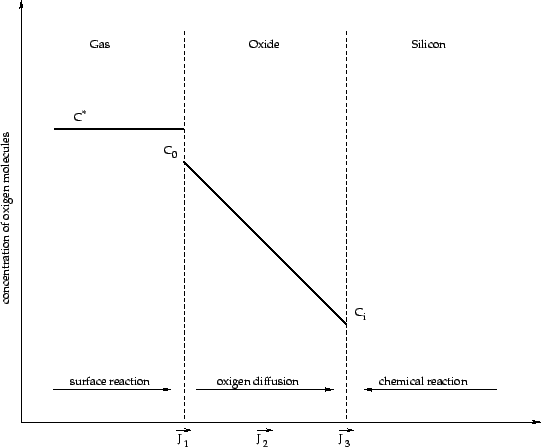At first, each of these steps can be described as independent flux equation, where the surface reaction can be written as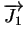= h1 . (C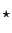- C0) (2.25)

where Crepresents the concentration of oxygen in the surrounding gas, C0 the concentration at the oxide interface and h1 a surface transport coefficient.

The diffusion is handled after Fick's law described in Chapter 2.1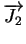= - D . grad (C) (2.26)

and finally the chemical reaction at the silicon/ silicon dioxide interface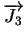= h2 . Ci (2.27)

with h2 as reaction ratio of the oxidation process. Thus, the oxide growth rate is equal to the rate of oxidizing molecules F reacting at the interface, divided by the number of oxide molecules N being incorporated to form a unit volume of oxide. Assuming equilibrium===the one-dimensional oxidation ratio can be written as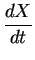=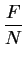(2.28)

where X denotes the oxide thickness. After eliminating Ci and C0 using (2.25), (2.26) and (2.27), a differential equation of the form=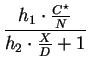=(2.29)

can be found with the parameters
 A = 2D .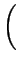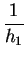+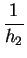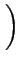(2.30) B = 2D .. (2.31)

The analytic solution of this differential equation with the start condition X = 0 at t = 0 is a quadratic equation of the form

 X2 + A . X = B . t        or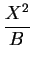+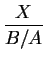= t. (2.32)

with the explicit result for the oxide thickness depending on time
 X =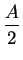.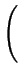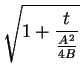- 1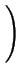(2.33)

Looking at the solution two different limiting values are recognizable. On the one hand, for long oxidation times ( tA2/4B) an approximately parabolic growth law
 X =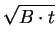(2.34)

can be established and, on the other hand, for very short oxidation times ( t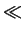A2/4B) a nearly linear trend of the oxidation growth with
 X =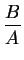. t (2.35)

can be assumed. Although the model of Deal & Grove was found in 1965 it was not possible to explain the parameters A and B on physical basics and, therefore, they are just known as fitting parameters. Furthermore, for very small oxide thickness sizes (X < 35 nm) the model does not fit the experimental data. Unfortunately, these sizes are of increasing importance since device sizes of transistors are dramatically shrinking. Nevertheless, in practice this model is more or less exclusively used to simulate the oxidation phenomena, where the parameters (Table 2.3) are often calculated after Arrhenius' law:
 B = B0 . e-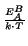(2.36)=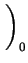. e-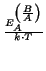(2.37)

Table 2.3: Experimental literature values for dry and wet oxidation parameters and their activation energies
 Type BA EAB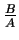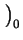EA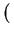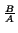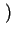Reference [nm/min] [eV] [nm2/min] [eV] wet 3.1 . 105 0.705 9.7 . 108 1.93 [Dea65] dry 2.2 . 108 1.286 1.28 . 108 2.02 [Dea65] dry - - 8.9 . 107 1.64 [dM72] dry 5.3 . 109 1.7 1.8 . 107 1.7 [Ire74] dry 2.6 . 1012 2.3 6 . 105 1.5 [Ire76] wet 7.0 . 107 ( > 950oC) 0.78 3.0 . 109 ( > 900oC) 2.05 [Lie81] wet 2.8 . 107 ( < 950oC) 1.17 3.5 . 107 ( < 900oC) 2.05 [Lie81] dry 7.35 . 106 ( < 1000oC) 1.76 1.70 . 1011 ( < 1000oC) 2.20 [Sch91] dry 7.35 . 108 ( > 1000oC) 2.25 5.79 . 106 ( > 1000oC) 2.20 [Sch91] wet 9.92 . 108 2.01 3.36 . 108 ( < 950oC) 1.20 [Sch91] wet 9.92 . 108 2.01 5.12 . 106 ( > 950oC) 0.76 [Sch91] dry 1.038 . 108 2.0 12.87 . 103 1.23 [TMA97] wet 3.450 . 107 ( < 900oC) 1.6 2.83 . 105 ( < 950oC) 1.17 [TMA97] wet 2.950 . 109 ( > 900oC) 2.05 7.00 . 103 ( > 950oC) 0.78 [TMA97]

Since the parameter values are varying in a wide range, and no physical description was found, a lot of tries to find an adequate model for oxidation have been made during the last 30 years, but till now with limited success.Next: 2.2.2 Point Defect Models Up: 2.2 Oxidation Previous: 2.2 Oxidation